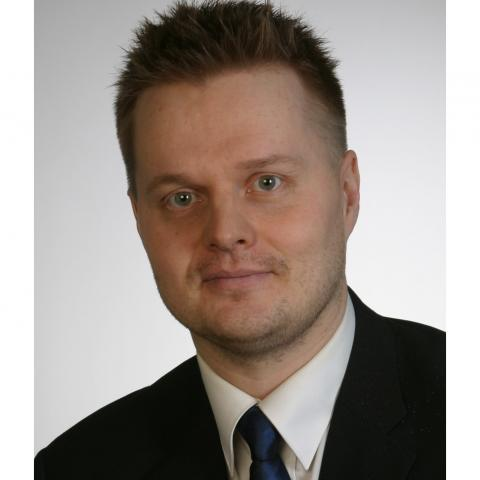# Jukka Kemppainen

PhD
Lecturer
Applied Mathematics

My research is related to integro-differential equations, which means that the equations contain derivatives and integrals. More precisely, my research is related to the partial differential equations having non-integer order derivatives, which have non-local character. The non-local character of the equations bring some new difficulties and new phenomena, which are not seen in the context of the classical partial differential equations. I have studied different aspects of non-local in time partial differential equations including existence and uniqueness of the solution and long-time behavior of the solutions.

My teaching consists of the basic mathematics courses aimed mainly for the engineering students. At the moment I am lecturing the courses Complex Analysis and Probability and Mathematical Statistics.

## Researcher information

### Researcher profiles## Contact information

### E-mail

jukka.t.kemppainen@oulu.fi

0294 482667